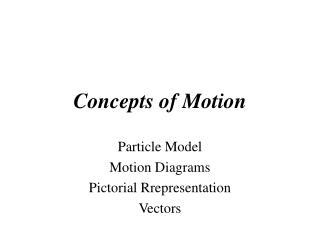Download PresentationConcepts of Motion# Concepts of Motion - PowerPoint PPT Presentation

Download Presentation##### Concepts of Motion

Download Policy: Content on the Website is provided to you AS IS for your information and personal use and may not be sold / licensed / shared on other websites without getting consent from its author. While downloading, if for some reason you are not able to download a presentation, the publisher may have deleted the file from their server.

- - - - - - - - - - - - - - - - - - - - - - - - - - - E N D - - - - - - - - - - - - - - - - - - - - - - - - - - -
##### Presentation Transcript

1. Concepts of Motion Particle Model Motion Diagrams Pictorial Rrepresentation Vectors

2. Reading Question For class today I read • Zero per cent • 20 per cent • 40 per cent • 60 per cent • 80 per cent • 100 per cent

3. Reading Question I did not tell a lie on the last question • True • False

4. Reading Question What is a “particle”? 1. Any part of an atom 2. An object that can be represented as a mass at a single point in space 3. A part of a whole 4. An object that can be represented as a single point in time 5. An object that has no top or bottom, no front or back

5. Reading Question What is a “particle”? 1. Any part of an atom 2. An object that can be represented as a mass at a single point in space 3. A part of a whole 4. An object that can be represented as a single point in time 5. An object that has no top or bottom, no front or back

6. Reading Question What quantities are shown on a complete motion diagram? 1. The position of the object in each frame of the film, shown as a dot 2. The average velocity vectors (found by connecting each dot in the motion diagram to the next with a vector arrow) 3. The average acceleration vectors (there is one acceleration vector linking each two velocity vectors) 4. All of the above

7. Reading Question What quantities are shown on a complete motion diagram? 1. The position of the object in each frame of the film, shown as a dot 2. The average velocity vectors (found by connecting each dot in the motion diagram to the next with a vector arrow) 3. The average acceleration vectors (there is one acceleration vector linking each two velocity vectors) 4. All of the above

8. Reading Question The pictorial representation of a physics problem consists of 1. a sketch. 2. a coordinate system. 3. symbols. 4. a table of values. 5. all of the above.

9. Reading Question The pictorial representation of a physics problem consists of 1. a sketch. 2. a coordinate system. 3. symbols. 4. a table of values. 5. all of the above.

10. Vectors A vector is defined as a mathematical quantity with magnitude and direction. • displacement • temperature • velocity • pressure • acceleration A scalar is a mathematical quantity that has magnitude only

11. Vectors

13. Vectors Vector Properties

14. Vectors Displacement

15. Class Question A particle moves from position 1 to position 2 during the interval ∆t. Which vector shows the particle’s average velocity? (1) (2) (3) (4) (5)

16. Class Question A particle moves from position 1 to position 2 during the interval ∆t. Which vector shows the particle’s average velocity? (1) (2) (3) (4) (5)

17. Class Question A particle undergoes acceleration while moving from point 1 to point 2. Which of the choices shows the velocity vector as the object moves away from point 2? (1) (3) (2) (4)

18. Class Question A particle undergoes acceleration while moving from point 1 to point 2. Which of the choices shows the velocity vector as the object moves away from point 2? (1) (3) (4) (2)

19. Class Question An acceleration vector 1. tells you how fast an object is going. 2. is constructed from two velocity vectors. 3. is the second derivative of the position. 4. is parallel or opposite to the direction of motion. 5. Acceleration vectors weren’t discussed in this chapter.

20. Class Question An acceleration vector 1. tells you how fast an object is going. 2. is constructed from two velocity vectors. 3. is the second derivative of the position. 4. is parallel or opposite to the direction of motion. 5. Acceleration vectors weren’t discussed in this chapter.